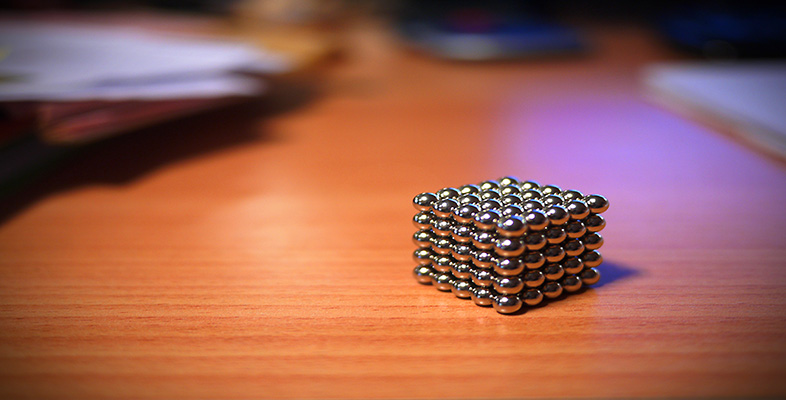Squares, roots and powers

Start this free course now. Just create an account and sign in. Enrol and complete the course for a free statement of participation or digital badge if available.

Free course

# 2.4.1 Try some yourself

## Activity 25

Write the following as a number to a single power:

• (a) 26 ÷ 22

• (b) 1010 ÷ 107

• (c) 78 ÷ 74

• (a) 26 ÷ 22 = 2(6 − 2) = 24

• (b) 1010 ÷ 107 = 10(10 − 7) = 103

• (c) 78 ÷ 74 = 7(8 − 4) = 74

## Activity 26

Express as powers of ten

• (a) a million

• (b) a thousand

• (c) a million divided by a thousand

• (a) a million = 1 000 000 = 106

• (b) a thousand = 1 000 = 103

• (c) 106 divided by 103 = 106–3 = 103 = a thousand

MU120_4M4